# NCERT Solutions (Ex: 5.1 to 5.3): Understanding Elementary Shapes Notes | Study Mathematics (Maths) Class 6 - Class 6

## Class 6: NCERT Solutions (Ex: 5.1 to 5.3): Understanding Elementary Shapes Notes | Study Mathematics (Maths) Class 6 - Class 6

The document NCERT Solutions (Ex: 5.1 to 5.3): Understanding Elementary Shapes Notes | Study Mathematics (Maths) Class 6 - Class 6 is a part of the Class 6 Course Mathematics (Maths) Class 6.
All you need of Class 6 at this link: Class 6

Exercise 5.1
Ques 1: What is the disadvantage in comparing line segments by mere observation?
Ans: There may be chance of error due to improper viewing.

Ques 2: Why is it better to use a divider than a ruler, while measuring the length of a line segment?
Ans: It is better to use a divider than a ruler, because the thickness of the ruler may cause difficulties in reading off her length. However divider gives up accurate measurement.

Ques 3: Draw any line segment, say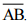Take any point C lying in between A and B. Measure the lengths of AB, BC and AC. Is AB = AC + CB?
[Note: If A, B, C are any three points on a line, such that AC + CB = AB, then we can be sure that C lies between A and B.]
Ans: Yes.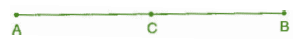AB = 6.5 cm, AC = 3cm, CB = 3.5 cm
AC + CB = 3 cm + 3.5 cm = 6.5 cm = AB

Ques 4: If A, B, C are three points on a line such that AB = 5 cm, BC = 3cm and AC = 8 cm, which one of them lies between the other two?
Ans: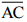is the longest line segment, thus B is the point between A and C.

Ques 5: Verify whether D is the mid-point of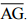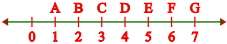Ans: AD = 3 units, DG = 3 units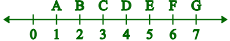Thus, D is the mid-point.

Ques 6: If B is the mid-point of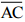and C is the mid-point of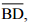where A, B, C, D lie on a straight line, say why AB = CD?
Ans: B is the mid-point of.
∴ AB = BC   ... (i)
And C is the mid-point of.
∴ BC = CD  ... (ii)
From equation (i) and (ii), we get
AB = CD

Ques 7: Draw five triangles and measure their sides. Check in each case, of the sum of the lengths of any two sides is always less than the third side.
Ans: Yes, sum of two sides of a triangle is always greater than the third side.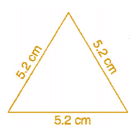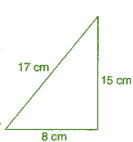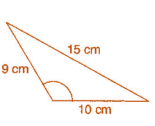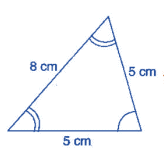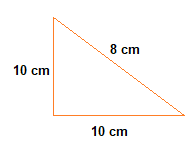Exercise 5.2
Ques 1: What fraction of a clockwise revolution does the hour hand of a clock turn through, when it goes from
(a) 3 to 9
(b) 4 to 7
(c) 7 to 10
(d) 12 to 9
(e) 1 to 10
(f) 6 to 3
Ans: (a) 1/2 or two right angles.
(b) 1/4 or one right angle.
(c) 1/4 or one right angle.
(d) 3/4 or three right angles.
(e) 3/4 or three right angles.
(f) 3/4 or three right angles.

Ques 2: Where will the hand of a clock stop if it:
(a) starts at 12 and make 1/2 of a revolution, clockwise?
(b) starts at 2 and makes 1/2 of a revolution, clockwise?
(c) starts at 5 and makes 1/4 of a revolution, clockwise?
(d) starts at 5 and makes 3/4 of a revolution, clockwise?
Ans: (a) At 6
(b) At 8
(c) At 8
(d) At 2

Ques 3: Which direction will you face if you start facing:
(a) East and make 1/2 of a revolution clockwise?
(b) East and make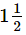of a revolution clockwise?
(c) West and makes 3/4 of a revolution, clockwise?
(d) South and make one full revolution?
(Should we specify clockwise or anti-clockwise for this last question? Why not?)
Ans: (a) West
(b) West
(c) North
(d) South
(For answer (d), it is immaterial whether we turn clockwise or anticlockwise, because one full revolution will bring us back to the original position)

Ques 4: What part of a revolution have you turned through if you stand facing:
(a) East and turn clockwise to face north?
(b) South and turn clockwise to face east?
(c) West and turn clockwise to face east?
Ans: (a) 3/4
(b) 3/4
(c) 1/2

Ques 5: Find the number of right angles turned through by the hour hand of a clock when it goes from:
(a) 3 to 6
(b) 2 to 8
(c) 5 to 11
(d) 10 to 1
(e) 12 to 9
(f) 12 to 6
Ans: (a) One right angle
(b) Two right angles
(c) Two right angles
(d) One right angle
(e) Three right angles
(f) Two right angles

Ques 6: How many right angles do you make if you start facing:
(a) South and turn clockwise to west?
(b) North and turn anti-clockwise to east?
(c) West and turn to west?
(d) South and turn to north?
Ans: (a) One right angle
(b) Three right angles
(c) Four right angles
(d) Two right angles

Ques 7: Where will the hour hand of a clock stop if it starts:
(a) from 6 and turns through 1 right angle?
(b) from 8 and turns through 2 right angles?
(c) from 10 and turns through 3 right angles?
(d) from 7 and turns through 2 straight angles?
Ans: (a) At 9
(b) At 2
(c) At 7
(d) At 7

Exercise 5.3
Ques 1: Match the following:

 (i) Straight angle (a) less than one-fourth a revolution (ii) Right angle (b) more than half a revolution (iii) Acute angle (c) half of a revolution (iv) Obtuse angle (d) one-fourth a revolution (v) Reflex angle [e] between 1/4 and 1/2 of a revolution (f) one complete revolution

Ans:
(i) → (c)
(ii) → (d)
(iii) → (a)
(iv) → (e)
(v) → (b)

Ques 2: Classify each one of the following angles as right, straight, acute, obtuse or reflex: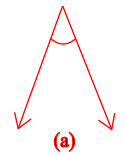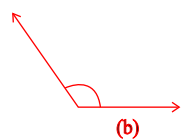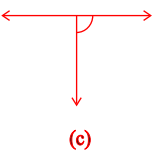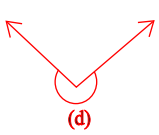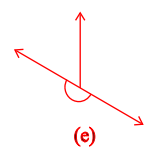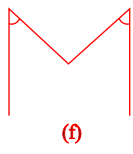Ans: (a) Acute angle
(b) Obtuse angle
(c) Right angle
(d) Reflex angle
(e) Straight angle
(f) Acute angle

For practicing more exercises of NCERT of the chapter "Understanding Elementary Shapes", open the links given below:

The document NCERT Solutions (Ex: 5.1 to 5.3): Understanding Elementary Shapes Notes | Study Mathematics (Maths) Class 6 - Class 6 is a part of the Class 6 Course Mathematics (Maths) Class 6.
All you need of Class 6 at this link: Class 6Use Code STAYHOME200 and get INR 200 additional OFF

## Mathematics (Maths) Class 6

239 videos|259 docs|43 tests

Track your progress, build streaks, highlight & save important lessons and more!

,

,

,

,

,

,

,

,

,

,

,

,

,

,

,

,

,

,

,

,

,

;